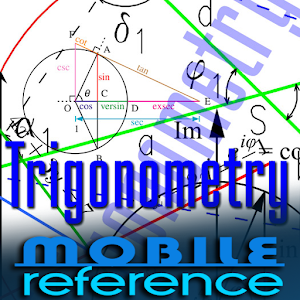# Trigonometry Study Guide - Lumos Educational App Store31
Price - \$NA

#### DESCRIPTION:

Features Clear and concise explanations Difficult concepts are explained in simple terms Illustrated with graphs and diagrams Search for the words or phrases Access the guide anytime, anywhere - at home, on the train, in the subway. Use your down time to prepare for an exam. Always have the guide available for a quick reference. Table of Contents Introduction: Overview | Basic definitions | Extending the definitions | Mnemonics | Pi | Radian Trigonometric Function Definitions: Right triangle definitions | Unit-circle definitions Trigonometric Identities: Notation | Periodici

#### OVERVIEW:

Trigonometry Study Guide is a free educational mobile app By MobileReference.It helps students in grades HS practice the following standards HSF.TF.A.1,HSF.TF.A.2,HSF.TF.A.3.

This page not only allows students and teachers download Trigonometry Study Guide but also find engaging Sample Questions, Videos, Pins, Worksheets, Books related to the following topics.

1. HSF.TF.A.1 : Understand radian measure of an angle as the length of the arc on the unit circle subtended by the angle.

2. HSF.TF.A.2 : Explain how the unit circle in the coordinate plane enables the extension of trigonometric functions to all real numbers, interpreted as radian measures of angles traversed counterclockwise around the unit circle.

3. HSF.TF.A.3 : Use special triangles to determine geometrically the values of sine, cosine, tangent for π/3, π/4 and π/6, and use the unit circle to express the values of sine, cosine, and tangent for x, π + x, and 2π - x in terms of their values for x, where x is any real number..

HS

#### STANDARDS:

HSF.TF.A.1
HSF.TF.A.2
HSF.TF.A.3
HSF.TF.A.4
HSF.TF.B.5
HSF.TF.B.6
HSF.TF.B.7
HSF.TF.C.8
HSF.TF.C.9

Developer: MobileReference

Software Version: 12.2

Category: Education

### RELATED APPSEdSearch WebSearch